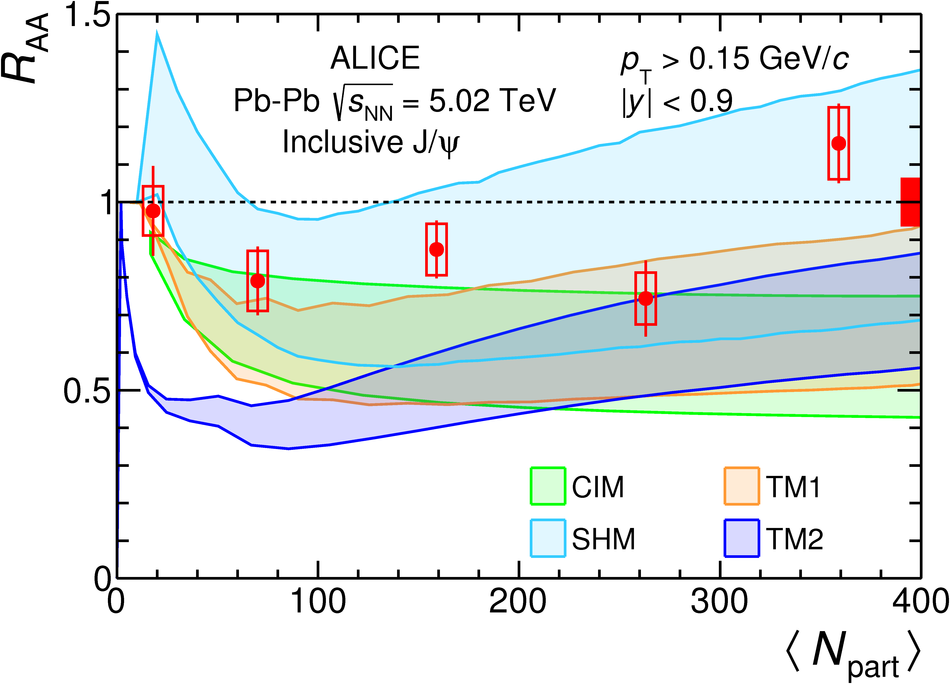# Figure 6

 Inclusive $\jpsi$ nuclear modification factor at midrapidity, integrated over $\pt$, as a function of $\langle\npart\rangle$ in $\PbPb$ collisions at $\snn = 5.02$ TeV compared with results at $\snn = 2.76$ TeV  (left panel) and with calculations from the CIM , SHM , TM1  and TM2  models (right panel). The yields in the left panel are shown without the low-$\pt$ cut-off in order to be able to compare with the lower energy data which are obtained for $\pt>0$. The calculations are shown as bands indicating the model uncertainties. Boxes around unity at $\Npart \approx 400$ in both panels indicate the correlated uncertainty of the data points due to the cross section in $\pp$ collisions.